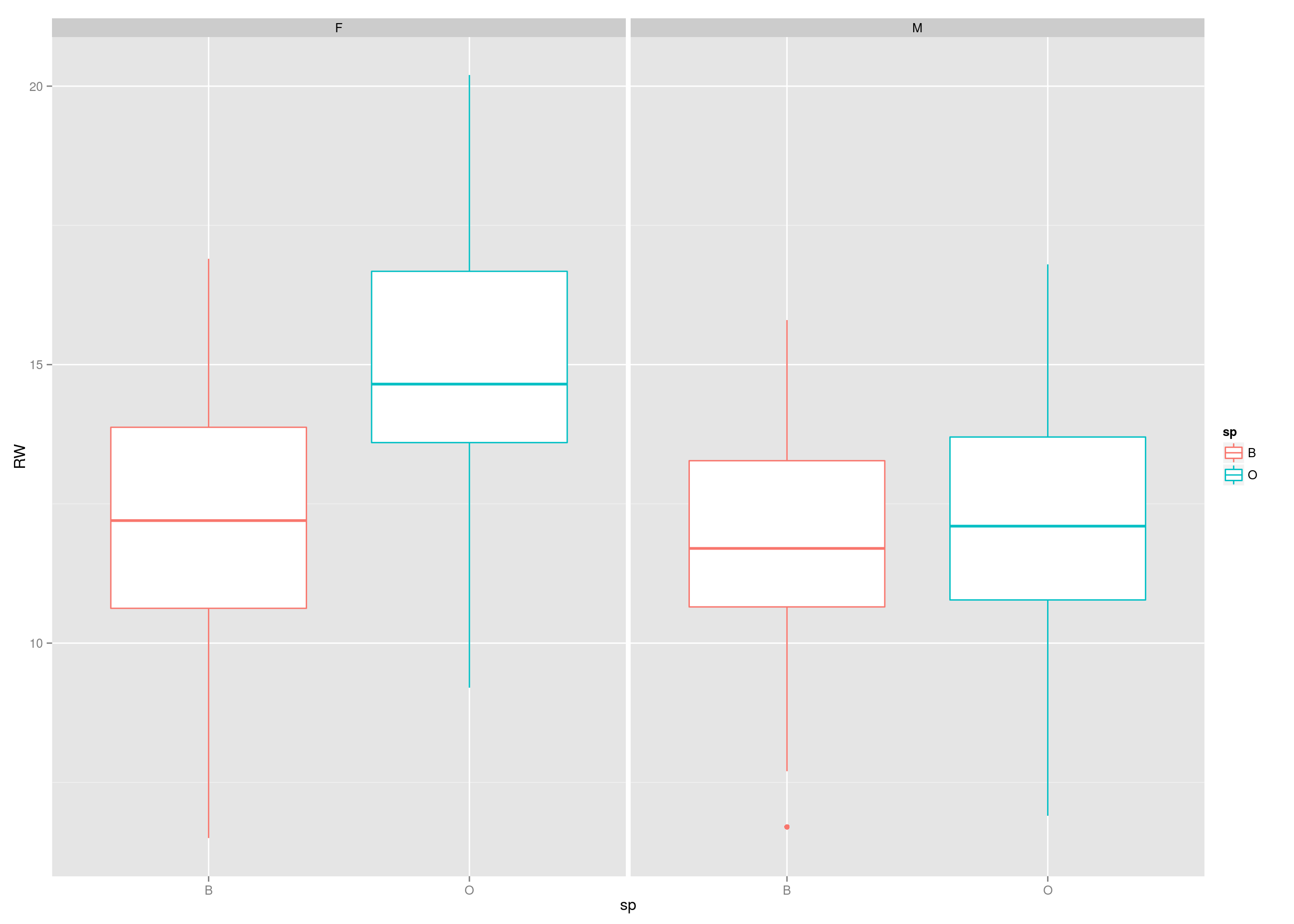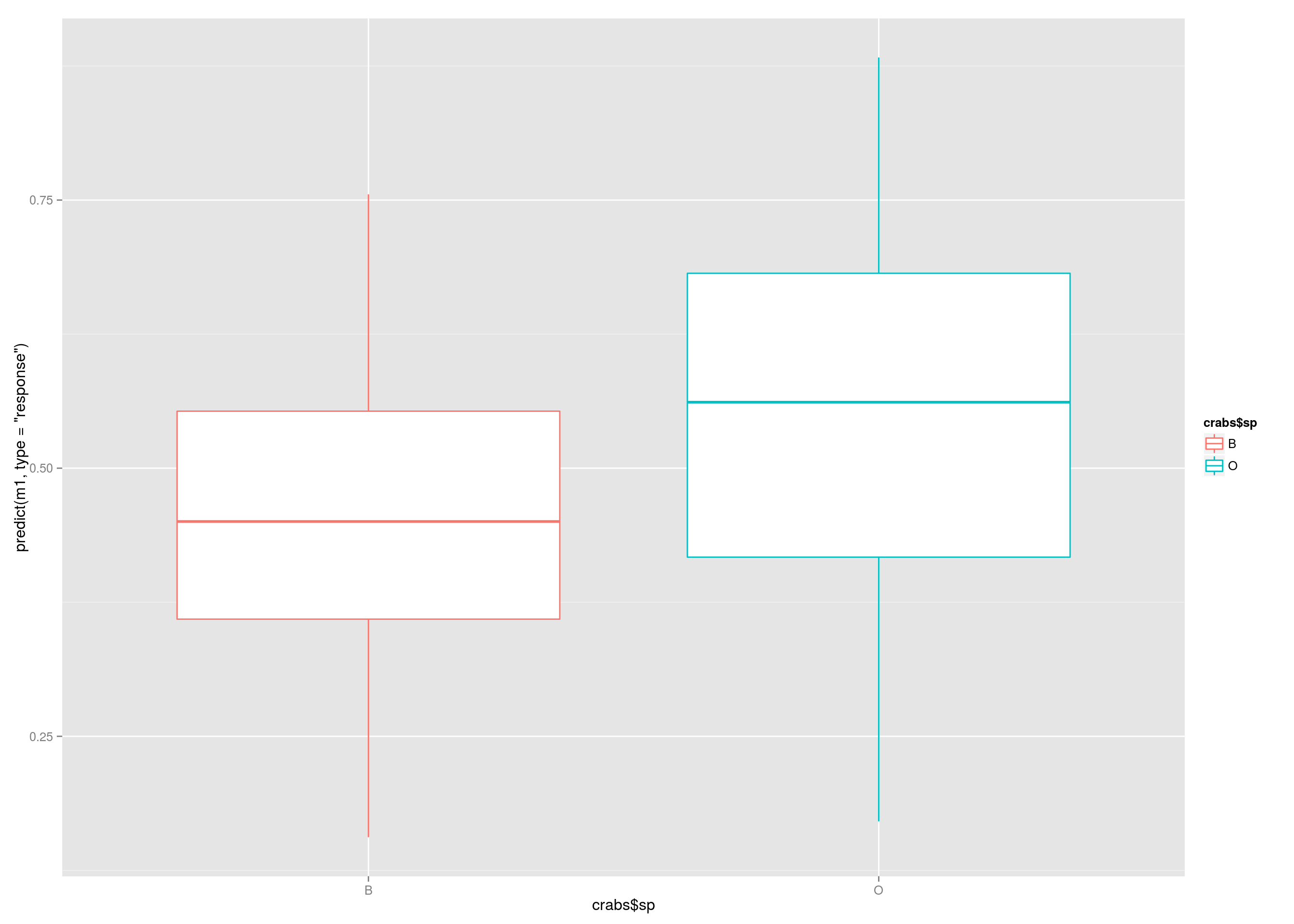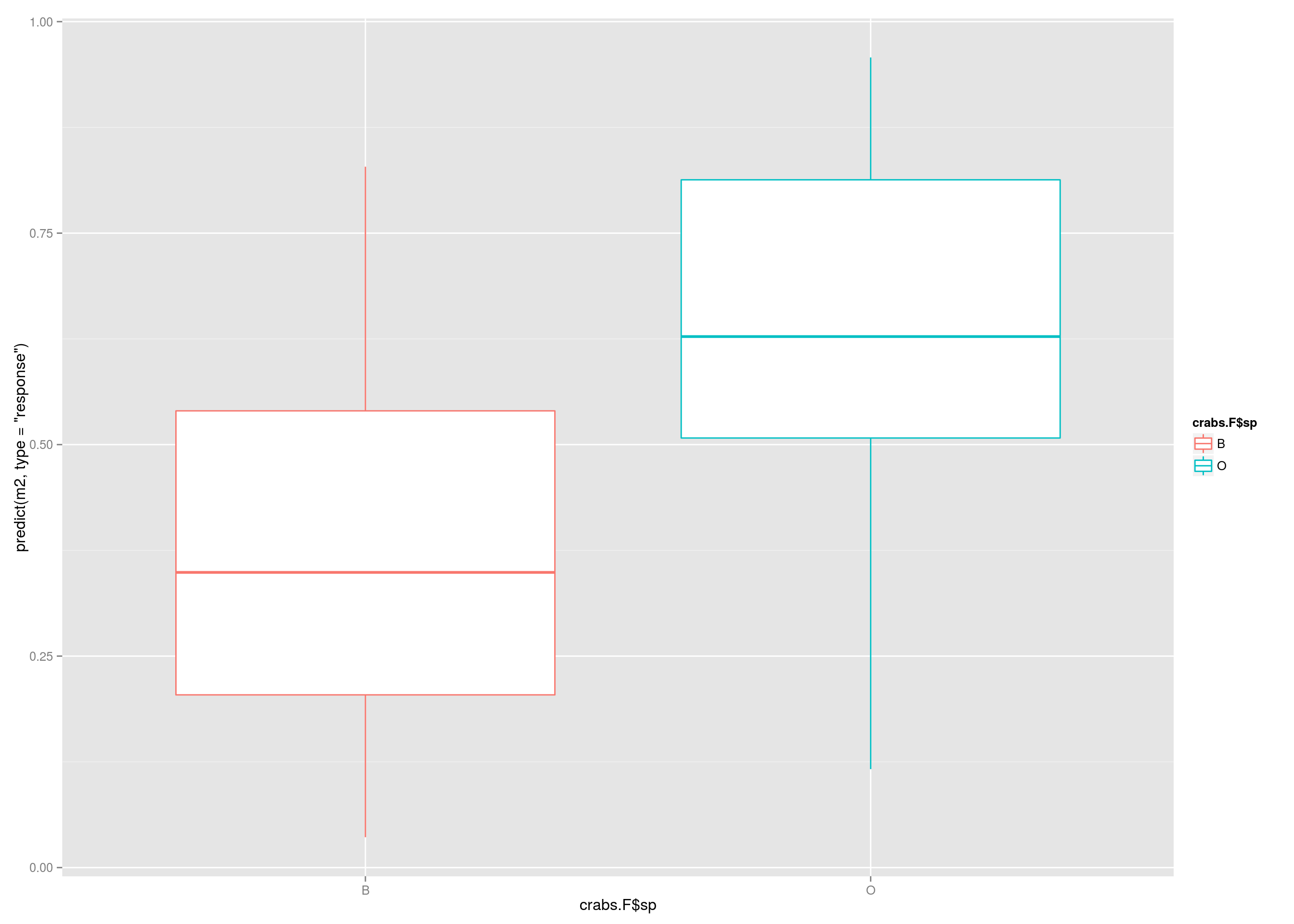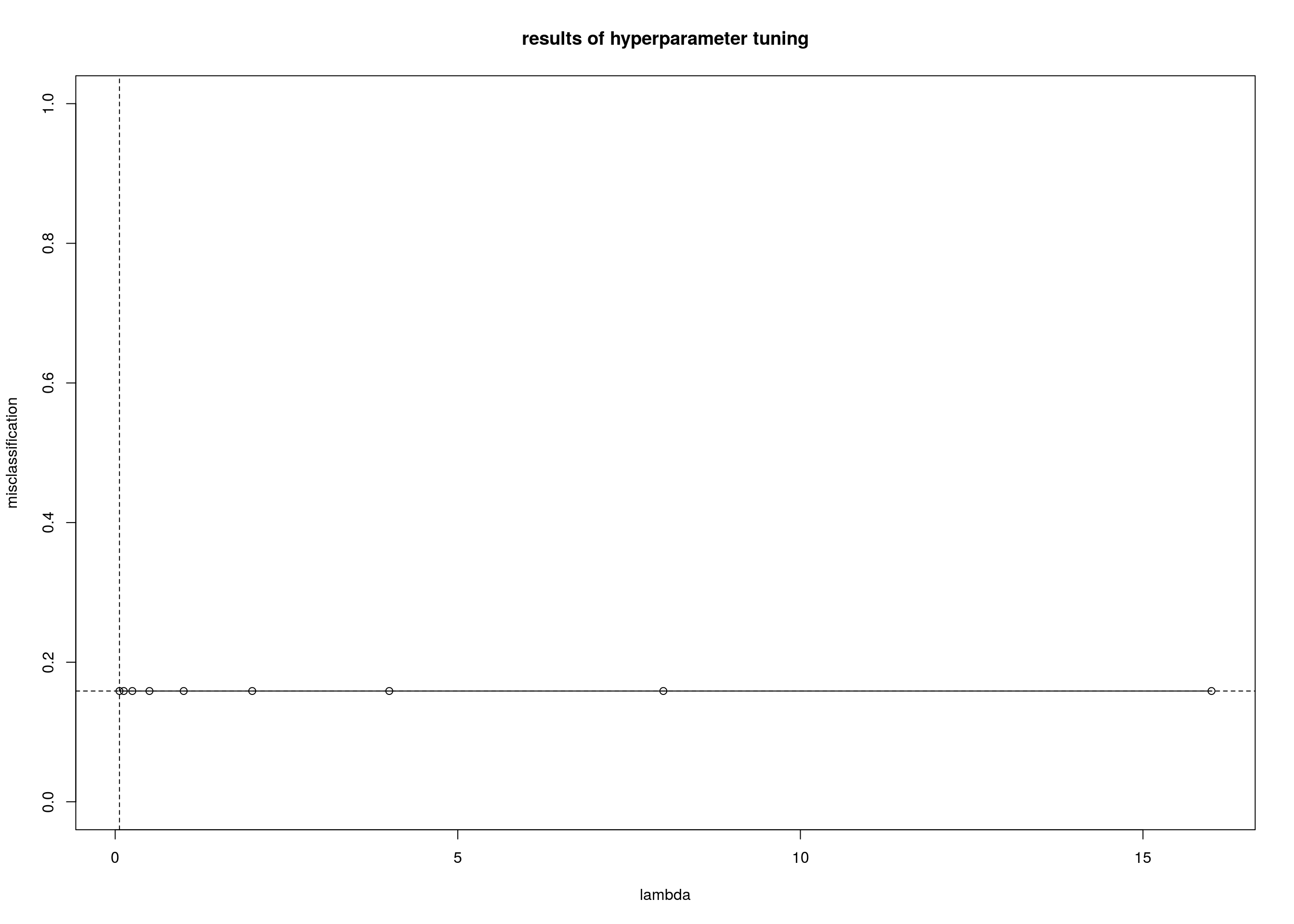Bernd Klaus, based on material form S. Arora (Bioc Seattle, Oct 14) and VJ Carey (Brixen 2011) as well as the CMA package vignete.

 European Molecular Biology Laboratory (EMBL), Heidelberg, Germany.

LAST UPDATE AT

Thu Feb 5 16:40:16 2015

## Preparations

We first set global chunk options and load the neccessary packages.

library(BiocStyle)
library(rmarkdown)
library(geneplotter)
library(ggplot2)
library(plyr)
library(dplyr)
library(LSD)
library(DESeq2)
library(gplots)
library(RColorBrewer)
library(stringr)
library(topGO)
##
## groupGOTerms:    GOBPTerm, GOMFTerm, GOCCTerm environments built.
library(genefilter)
library(biomaRt)
library(classifly)
library(MASS)
library(EDASeq)
library(fdrtool)
library(e1071)
library(LearnBioconductor)
## get it  here:
#http://bioconductor.org/help/course-materials/2014/SeattleOct2014/LearnBioconductor_0.1.6.tar.gz
library(GenomicRanges)
library(CMA)
library(plsgenomics)
library(crossval)
library(MLInterfaces)
library(LSD)
library(GenomicRanges)
library(GGally)
library(MLSeq)
library(caret)
library(dendextend)
library(class)
library(rpart)
library(ALL)

#library(rggobi)

#library(xlsx)

In this document we introduce some ML examples.

# Introduction to Machine Learning

The term machine learning refers to a family of computational methods for analyzing multivariate datasets. Each data point has a vector of features in a shared feature space, and may have a class label from some fixed finite set. Supervised learning refers to processes that help articulate rules that map feature vectors to class labels. The class labels are known and function as supervisory information to guide rule construction. Unsupervised learning refers to processes that discover structure in collections of feature vectors. Typically the structure consists of a grouping of objects into clusters. This practical introduction to machine learning will begin with a survey of a low- dimensional dataset to fix concepts, and will then address problems coming from genomic data analysis, using RNA expression and chromatin state data.

Statistical Learning plays an important role in Genomics. A few example of learning problems would be

• Identify the risk factors(genes) for prostrate cancer based on gene expression data

• Predict the chances of breast cancer survival in a patient.

• Identify patterns of gene expression among different sub types of breast cancer

Typically, we have an outcome measurement, usually quantitative (such as gene expression) or categorical (such as breast cancer /no breast cancer), that we wish to predict based on a set of features (such as diet and clinical measurements). We have a training set of data, in which we observe the outcome and feature measurements for a set of objects (such as people). With this data we build a prediction model, or a learner, which will enable us to predict the outcome for new unseen objects. A good learner is one that accurately predicts such an outcome. This is called supervised learning because the outcome variable guides the learning process. In the unsupervised learning problem, have no measurements of the outcome and observe only the features. Our task is to describe how the data are organized or clustered.

# Getting acquainted with machine learning via the crabs data

The crabs data will be used to introduce you to machine learning methods. The crabs data frame has 200 rows and 8 columns, describing 5 morphological measurements on 50 crabs each of two color forms and both sexes, of the species Leptograpsus variegatus collected at Fremantle, W. Australia.

data("crabs")
dim(crabs)
##  200   8
sample_n(crabs,10)
##     sp sex index   FL   RW   CL   CW   BD
## 68   B   F    18 12.0 10.7 24.6 28.9 10.5
## 15   B   M    15 12.8 10.9 27.4 31.5 11.0
## 157  O   F     7 14.0 12.8 28.8 32.4 12.7
## 30   B   M    30 16.1 11.6 33.8 39.0 14.4
## 179  O   F    29 18.4 15.7 36.5 41.6 16.4
## 20   B   M    20 13.9 11.1 29.2 33.3 12.1
## 196  O   F    46 21.4 18.0 41.2 46.2 18.7
## 3    B   M     3  9.2  7.8 19.0 22.4  7.7
## 111  O   M    11 14.0 11.5 29.2 32.2 13.1
## 117  O   M    17 14.2 11.3 29.2 32.2 13.5
table(crabs$sex) ## ## F M ## 100 100 We can plot the rear width (RW, in mm) per sex and species to get on idea on whether it differs by age group. qplot(sp, RW, data = crabs, facets = ~ sex, color = sp, geom = "boxplot")We will regard these data as providing five quantitative features (FL, RW, CL, CW, BD) 1 and a pair of class labels (sex, sp=species). We may regard this as a four class problem, or as two two class problems. Our first problem does not involve any computations. If you want to write R code to solve the problem, do so, but use prose first. • Question On the basis of the boxplots in the Fiugre, comment on the prospects for predicting species on the basis of RW. State a rule for computing the predictions. Describe how to assess the performance of your rule. ## Species prediction via logistic regression A simple approach to prediction involves logistic regression, which uses a logit transformation of the class labels and then puts in the predictors in a regresssion curve. m1 = glm(sp~RW, data=crabs, family=binomial) summary(m1) ## ## Call: ## glm(formula = sp ~ RW, family = binomial, data = crabs) ## ## Deviance Residuals: ## Min 1Q Median 3Q Max ## -1.6781 -1.0884 -0.0417 1.0716 1.8803 ## ## Coefficients: ## Estimate Std. Error z value Pr(>|z|) ## (Intercept) -3.4491 0.8221 -4.20 0.000027 *** ## RW 0.2708 0.0635 4.27 0.000020 *** ## --- ## Signif. codes: 0 '***' 0.001 '**' 0.01 '*' 0.05 '.' 0.1 ' ' 1 ## ## (Dispersion parameter for binomial family taken to be 1) ## ## Null deviance: 277.26 on 199 degrees of freedom ## Residual deviance: 256.35 on 198 degrees of freedom ## AIC: 260.4 ## ## Number of Fisher Scoring iterations: 4 The logistic regression fit will tell us the probability for sample of it belonging to class O. Below, we classify a sample into the O class if the probability for it is greater than 0.5. • Question Write down the statistical model corresponding to the R expression above. How can we derive a classifier from this model? • Question Perform the following computations. Discuss their interpretation. What are the estimated error rates of the two models? Is the second model, on the subset, better? qplot(crabs$sp, predict(m1,type="response"), geom  = "boxplot", color=crabs$sp )table(predict(m1,type="response")>.5, crabs$sp)
##
##          B  O
##   FALSE 62 37
##   TRUE  38 63
crabs.F <- subset(crabs, sex=="F")
m2  = glm(sp~RW, data=crabs.F, family=binomial)

table(predict(m2,type="response")>.5, crabs.F$sp) ## ## B O ## FALSE 34 12 ## TRUE 16 38 qplot(crabs.F$sp, predict(m2,type="response"), geom  = "boxplot", color=crabs.F$sp )What we computed above is commonly called “confusion Matrix”: For a two–class scenario, it gives the number of true positives / negatives and the errors represented by false positives and false negatives. This terminology stems from medical disease testing, obviously, in our example there is no natural “positive” or “negative” category. Thus, we set (arbitrarly) “B” as negative. cMatrix = crossval::confusionMatrix(actual = crabs.F$sp,
predicted = ifelse(predict(m2,type="response")>.5, "O", "B"),
negative="B")

cMatrix 
## FP TP TN FN
## 16 38 34 12
## attr(,"negative")
##  "B"
diagnosticErrors(cMatrix)
##    acc   sens   spec    ppv    npv    lor
## 0.7200 0.7600 0.6800 0.7037 0.7391 1.9065
## attr(,"negative")
##  "B"

The function diagnosticErrors computes various diagnostic errors useful for evaluating the performance of a diagnostic test or a classifier: accuracy (acc), sensitivity (sens), specificity (spec), positive predictive value (ppv), negative predictive value (npv), and log-odds ratio (lor). The defintions are:

• acc = (TP+TN)/(FP+TN+TP+FN)

• sens = TP/(TP+FN)

• spec = TN/(FP+TN)

• ppv = TP/(FP+TP)

• npv = TN/(TN+FN)

• lor = $$log$$(TP$$*$$TN/(FN$$*$$FP))

The first model is very bad, there is hardly any predictive power, if we condition on the sex and use only the females, the predictions become better.

## The cross-validation concept

Cross–validation is a technique that is widely used for reducing bias in the estimation of predictive accuracy. If no precautions are taken, bias can be caused by overfitting a classification algorithm to a particular dataset; the algorithm learns the classification “by heart”, but performs poorly when asked to generalize it to new, unseen examples. Briefly, in cross–validation the dataset is deterministically partitioned into a series of training and test sets. The model is built for each training set and evaluated on the test set. The accuracy measures are averaged over this series of fits. Leave–one–out cross–validation consists of N fits, with N training sets of size N–1 and N test sets of size 1. First let us use MLearn from the MLInterfaces package to fit a single logistic model. MLearn requires you to specify an index set for training. We use c(1:30, 51:80) to choose a training set of size 60, balanced between two species (because we know the ordering of records). This procedure also requires you to specify a probability threshold for classification. We use a typical default of 0.5. If the predicted probability of being “O” exceeds 0.5, we classify to “O”, otherwise to “B”.

ml1 = MLearn( sp~RW, crabs.F, glmI.logistic(thresh=.5), c(1:30, 51:80),
family=binomial)
ml1
## MLInterfaces classification output container
## The call was:
## MLearn(formula = sp ~ RW, data = crabs.F, .method = glmI.logistic(thresh = 0.5),
##     trainInd = c(1:30, 51:80), family = binomial)
## Predicted outcome distribution for test set:
##  O
## 40
## Summary of scores on test set (use testScores() method for details):
##    Min. 1st Qu.  Median    Mean 3rd Qu.    Max.
##   0.755   0.886   0.980   0.935   0.992   1.000
cMatrix.TT = crossval::confusionMatrix(actual = ml1@testOutcomes,
predicted =ml1@testPredictions,
negative="B")
cMatrix.TT
## FP TP TN FN
## 20 20  0  0
## attr(,"negative")
##  "B"
diagnosticErrors(cMatrix.TT)
##  acc sens spec  ppv  npv  lor
##  0.5  1.0  0.0  0.5  NaN  NaN
## attr(,"negative")
##  "B"
• Question What does the report on ml1 tell you about predictions with this model? Can you reconcile this with the results in model m2? (Hint — non–randomness of the selection of the training set is a problem.)

Now we will illustrate cross-validation. First, we scramble the order of records in the ExpressionSet so that sequentially formed groups are approximately random samples.

We invoke the now the MLearn specifying a five–fold cross-validation.

smx1 = MLearn( sp~RW, crabs.F, glmI.logistic(thresh=.5),
xvalSpec(type = "LOG", niter = 5, balKfold.xvspec(5))
, family=binomial)

cMatrix.cv = crossval::confusionMatrix(actual = smx1@testOutcomes,
predicted =smx1@testPredictions,
negative="B")
cMatrix.cv
## FP TP TN FN
## 17 37 33 13
## attr(,"negative")
##  "B"
diagnosticErrors(cMatrix.cv)
##    acc   sens   spec    ppv    npv    lor
## 0.7000 0.7400 0.6600 0.6852 0.7174 1.7093
## attr(,"negative")
##  "B"

We can now compare the crossvalidation results to the ones obtained using the training set also for testing and using only one training–test split.

rbind(noSplit = diagnosticErrors(cMatrix),
oneSplit = diagnosticErrors(cMatrix.TT),
CV5 = diagnosticErrors(cMatrix.cv)
)
##           acc sens spec    ppv    npv   lor
## noSplit  0.72 0.76 0.68 0.7037 0.7391 1.906
## oneSplit 0.50 1.00 0.00 0.5000    NaN   NaN
## CV5      0.70 0.74 0.66 0.6852 0.7174 1.709
• Question. Define clearly the difference between models sml1 and smx1

# Applying classification to Microarray data

Classification methods have been very popular for microarray data, in order to identify e.g. signatures of cancer subtypes. Traditional methods often yield unsatisfactory results or are even inapplicable in the $$p \gg n$$ setting.

Hence, microarray studies have stimulated the development of new approaches and motivated the adaptation of known traditional methods to high-dimensional data.

One of the most commonly used algorithms for this purpose is called NSC or PAM. See the paper.

Moreover, model selection and evaluation of prediction rules proves to be highly difficult in this situation for several reasons. Firstly, the hazard of overfitting, which is common to all prediction problems, is increased by high dimensionality. Secondly, the usual evaluation scheme based on the splitting into learning and test data sets often applies only partially in the case of small samples. Lastly, modern classification techniques rely on the proper choice of hyperparameters whose optimization is highly computer-intensive, especially in the case of high-dimensional data.

## Learning with expression arrays

Here we will concentrate on ALL: acute lymphocytic leukemia, B-cell type.

### Phenotype reduction

We will identify expression patterns that discriminate individuals with BCR/ABL fusion in B-cell leukemia.

data("ALL")
bALL = ALL[, substr(ALL$BT,1,1) == "B"] fus = bALL[, bALL$mol.biol %in% c("BCR/ABL", "NEG")]
fus$mol.biol = factor(fus$mol.biol)
fus
## ExpressionSet (storageMode: lockedEnvironment)
## assayData: 12625 features, 79 samples
##   element names: exprs
## protocolData: none
## phenoData
##   sampleNames: 01005 01010 ... 84004 (79 total)
##   varLabels: cod diagnosis ... date last seen (21 total)
## featureData: none
## experimentData: use 'experimentData(object)'
##   pubMedIds: 14684422 16243790
## Annotation: hgu95av2
sample_n(pData(fus), 10)
##         cod  diagnosis  sex age BT remission                 CR    date.cr t(4;11) t(9;22)
## 57001 57001  1/29/1997    F  53 B3      <NA> DEATH IN INDUCTION       <NA>   FALSE   FALSE
## 25006 25006  3/18/2000 <NA>  NA B2        CR                 CR   5/8/2000      NA      NA
## 04010  4010 10/30/1997    F  18 B1        CR                 CR   1/7/1998   FALSE   FALSE
## 12026 12026  5/29/1998    M  39 B2       REF                REF       <NA>   FALSE    TRUE
## 20002 20002   5/9/1997    F  58 B2        CR                 CR  8/19/1997   FALSE    TRUE
## 26003 26003  2/18/1998    F  49 B4        CR                 CR  4/21/1998   FALSE   FALSE
## 65005 65005  7/20/1999    M  22 B2       REF                REF       <NA>   FALSE    TRUE
## 43001 43001 11/14/1996    M  41 B1        CR                 CR  1/30/1997   FALSE    TRUE
## 26001 26001  9/27/1997    M  21 B2        CR                 CR 12/11/1997      NA      NA
## 28042 28042  6/18/1999    M  18 B3        CR                 CR   8/9/1999      NA      NA
##       cyto.normal                citog mol.biol fusion protein  mdr   kinet   ccr relapse
## 57001        TRUE               normal      NEG           <NA>  NEG hyperd.    NA      NA
## 25006          NA                 <NA>      NEG           <NA>  NEG    <NA>  TRUE   FALSE
## 04010       FALSE         complex alt.      NEG           <NA>  POS hyperd. FALSE    TRUE
## 12026       FALSE              t(9;22)  BCR/ABL      p190/p210 <NA> dyploid FALSE   FALSE
## 20002       FALSE        t(9;22)+other  BCR/ABL           p190  NEG dyploid FALSE    TRUE
## 26003       FALSE         del(p15/p16)  BCR/ABL           p210  NEG dyploid FALSE    TRUE
## 65005       FALSE t(9;22)+del(p15/p16)  BCR/ABL           p190  NEG dyploid    NA      NA
## 43001       FALSE              t(9;22)  BCR/ABL      p190/p210  POS dyploid FALSE    TRUE
## 26001          NA                 <NA>      NEG           <NA>  POS dyploid  TRUE   FALSE
## 28042          NA                 <NA>      NEG           <NA>  NEG dyploid FALSE    TRUE
##       transplant         f.u date last seen
## 57001         NA        <NA>           <NA>
## 25006      FALSE         CCR       3/3/2002
## 04010      FALSE         REL       3/5/1998
## 12026      FALSE DEATH IN CR           <NA>
## 20002      FALSE         REL     12/15/1997
## 26003      FALSE         REL       7/1/1998
## 65005         NA        <NA>           <NA>
## 43001      FALSE         REL      6/28/1998
## 26001      FALSE         CCR      7/31/2002
## 28042      FALSE         REL     11/30/2002

### Nonspecific filtering

We can nonspecifically filter to 300 genes (to save computing time) with largest measures of robust variation across all samples:

mads = apply(exprs(fus),1,mad)
fcol = ifelse(fusk$mol.biol=="NEG", "green", "red") ## Constructing a classification rule The second main issue is the construction of a appropriate prediction rule. In microarray data analysis, we have to deal with the $$n\ll p$$ situation, i.e. the number of predictors exceeds by far the number of observations. Some class prediction methods only work for the case $$n \ll p$$, e.g. linear or qudratic discriminant analysis which are based on the inversion of a matrix of size $$p\times p$$ and rank $$n-1$$. In the $$n\ll p$$ setting, the hazard of overfitting is especially acute: perfect separation of the classes for a given sample based on a high number of predictors is always possible. However, the resulting classification rule may generalize poorly on independent test data. There are basically three approaches to cope with the $$n\ll p$$ setting: • variable selection using, e.g., univariate statistical tests • regularization or shrinkage methods, such as the Support Vector Machine $$\ell_2$$ or $$\ell_1$$ penalized logistic regression or Boosting from which some also perform variable selection • dimension reduction or feature extraction. Most prominent is the Partial Least Squares method Most classification methods depend on a vector of hyperparameters $${\lambda}$$ that have to be correctly chosen. Together with variable selection, this is part of the model selection and has thus to be performed separately for each learning sample $${L}_b$$ to avoid bias. An optimal vector of hyperparameters $${\lambda}^{\text{opt}}$$ is determined by defining a discrete set of values whose performance is then measured by cross-validation. This involves a further splitting step, which is sometimes called ‘inner loop’ or ‘nested’ cross-validation. More precisely, each learning set $${L}_b$$ is divided into $$l=1,\ldots,k$$ parts such that the $$l$$-th part forms the test sample for the $$l$$-th (inner) iteration. This procedure can be used to derive an error estimator for each value on the grid of candiate hyperparameter values. The optimal vector $${\lambda}^{\text{opt}}$$ is chosen to minimize this error criterion. Note that there are often several such minimizers. Furthermore, the minimizer found by this procedure can be relatively far away from the true minimizer, depending on how fine the discrete grid has been chosen. The choice of the inner cross-validation scheme is difficult. With a high $$k$$, computation times soon become prohibitively high. With a low $$k$$, the size of $${L}_{b_l}, \; l=1,\ldots,k$$ is strongly reduced compared to the complete sample $$S$$, which may have an impact on the derived optimal parameter values. Nevertheless, nested cross-validation is commonly used in this context. Considering the computational effort required for hyperparameter optimization and the small sample sizes, one may prefer class prediction methods that do not depend on many hyperparameters and/or behave robustly against changes of the hyperparameter values. ## Using Logistic regression We first generate the learning sets for CV and then tune the parameters of the method. We use the method of Zhu and Hastie, 2004. labs <- pData(fus)$mol.biol
fiveCVdat <- GenerateLearningsets(y=labs,
method="CV", fold = 5, strat = TRUE)
fiveCVdat
## learningset mode:  stratified CV
## number of learningsets:  5
## (maximum) number of observations per learning set:  64
#?plrCMA

## tune the parameters
tuningstep <- CMA::tune(X = t(exprs(fus)), y=labs,
learningsets = fiveCVdat, classifier = "plrCMA")
##  "plrCMA"
## attr(,"package")
##  "CMA"
## tuning iteration 1
## tuning iteration 2
## tuning iteration 3
## tuning iteration 4
## tuning iteration 5
tuningstep
## tuning for 'plr'
## hyperparameters tuned:
## lambda
## CV folds used: 3
plot(tuningstep, iter = 3)unlist(CMA::best(tuningstep))
## lambda lambda lambda lambda lambda
## 0.0625 0.0625 0.0625 0.0625 0.0625
## run the classifier
class_fiveCV <- classification(X =  t(exprs(fus)), y=labs, classifier = "plrCMA",
learningsets = fiveCVdat, lambda=0.0625)
## iteration 1
## iteration 2
## iteration 3
## iteration 4
## iteration 5
##evaluate
av_logR <- evaluation(class_fiveCV, measure = "average probability",
scheme = "classwise", y=labs)
show(av_logR)
## evaluated method: 'plr'
## scheme used :'classwise'
## peformance measure: 'average probability'
## class-wise performance:
## BCR/ABL     NEG
##  0.6707  0.7679
#boxplot(av_logR)
#summary(av_logR)

## Using PAM

library(pamr)

pamTrain <- pamr.train(list(x= exprs(fus), y=labs))
## 123456789101112131415161718192021222324252627282930
pamTrain
## Call:
## pamr.train(data = list(x = exprs(fus), y = labs))
##    threshold nonzero errors
## 1  0.000     12625   14
## 2  0.202      8873   12
## 3  0.404      5858   12
## 4  0.605      3785   12
## 5  0.807      2469   10
## 6  1.009      1651   10
## 7  1.211      1123   9
## 8  1.412       778   9
## 9  1.614       546   7
## 10 1.816       385   7
## 11 2.018       275   7
## 12 2.220       201   7
## 13 2.421       151   7
## 14 2.623       115   7
## 15 2.825        86   7
## 16 3.027        59   7
## 17 3.229        35   6
## 18 3.430        26   5
## 19 3.632        20   6
## 20 3.834        15   7
## 21 4.036        11   7
## 22 4.237         9   9
## 23 4.439         7   9
## 24 4.641         7   9
## 25 4.843         5   10
## 26 5.045         4   12
## 27 5.246         2   12
## 28 5.448         2   20
## 29 5.650         2   37
## 30 5.852         0   37
#pam.results <- pamr.cv(pamTrain, list(x= exprs(fus), y=labs))

#predict
labs_new = pamr.predict(pamTrain, exprs(fus) , threshold=TRUE)

pred.err = sum(labs_new  != labs)/length(labs)
pred.err
##  0.1266
# class specific prediczion error
#pred.err = sum(labs_new  != labs & )/length(labs)

# Exploratory multivariate analysis

## Scatterplots

• Question 7 Interpret the following code, whose result is shown in Figure 2. Modify it to depict the pairwise configurations with different colors for crab genders.
pairs(crabs[,-c(1:3)], col=ifelse(crabs\$sp=="B", "blue", "orange"))